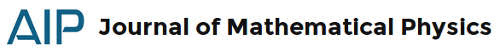### JMP 58, 2: quantum abstractI'm returning with the selections from the Journal of Mathematical Physics. I'm arranging two further post for this series for the next weeks. Stay tuned!
Alhaidari, A., & Taiwo, T. (2017). Wilson-Racah quantum system Journal of Mathematical Physics, 58 (2) DOI: 10.1063/1.4975138 (arXiv)
Using a recent formulation of quantum mechanics without a potential function, we present a four-parameter system associated with the Wilson and Racah polynomials. The continuum scattering states are written in terms of the Wilson polynomials whose asymptotics give the scattering amplitude and phase shift. On the other hand, the finite number of discrete bound states are associated with the Racah polynomials.
Dorsch, F. (2017). Accumulation rate of bound states of dipoles generated by point charges in strained graphene Journal of Mathematical Physics, 58 (2) DOI: 10.1063/1.4976201 (arXiv)
We consider strained graphene, modelled by the two-dimensional massive Dirac operator, with potentials corresponding to charge distributions with vanishing total charge, non-vanishing dipole moment and finitely many point charges of subcritical coupling constants located in the graphene sheet. We show that the bound state energies accumulate exponentially fast at the edges of the spectral gap by determining the leading order of the accumulation rate.Pandey, M., & Vaidya, S. (2017). Quantum phases of Yang-Mills matrix model coupled to fundamental fermions Journal of Mathematical Physics, 58 (2) DOI: 10.1063/1.4976503 (arXiv)
By investigating the $SU(2)$ Yang-Mills matrix model coupled to fundamental fermions in the adiabatic limit, we demonstrate quantum critical behaviour at special corners of the gauge field configuration space. The quantum scalar potential for the gauge field induced by the fermions diverges at the corners and is intimately related to points of enhanced degeneracy of the fermionic Hamiltonian. This in turn leads to superselection sectors in the Hilbert space of the gauge field, the ground states in different sectors being orthogonal to each other. As a consequence of our analysis, we show that 2-color quantum chromodynamics coupled to two Weyl fermions has three quantum phases. When coupled to a massless Dirac fermion, the number of quantum phases is four. One of these phases is the color-spin locked phase.
Holweck, F., Luque, J., & Thibon, J. (2017). Entanglement of four-qubit systems: A geometric atlas with polynomial compass II (the tame world) Journal of Mathematical Physics, 58 (2) DOI: 10.1063/1.4975098 (arXiv)We propose a new approach to the geometry of the four-qubit entanglement classes depending on parameters. More precisely, we use invariant theory and algebraic geometry to describe various stratifications of the Hilbert space by Stochastic Local Operations with Classical Communication (SLOCC) invariant algebraic varieties. The normal forms of the four-qubit classification of Verstraete et al. are interpreted as dense subsets of components of the dual variety of the set of separable states and an algorithm based on the invariants/covariants of the four-qubit quantum states is proposed to identify a state with a SLOCC equivalent normal form (up to qubits permutation).
Wei, A. (2017). On the numbers of images of two stochastic gravitational lensing models Journal of Mathematical Physics, 58 (2) DOI: 10.1063/1.4975139
We study two gravitational lensing models with Gaussian randomness: the continuous mass fluctuation model and the floating black hole model. The lens equations of these models are related to certain random harmonic functions. Using Rice’s formula and Gaussian techniques, we obtain the expected numbers of zeros of these functions, which indicate the amounts of images in the corresponding lens systems.
Llibre, J., & Tonon, D. (2017). Symmetric periodic orbits for the collinear charged 3-body problem Journal of Mathematical Physics, 58 (2) DOI: 10.1063/1.4974511 (sci-hub)
In this paper we study the existence of periodic symmetric orbits of the 3-body problem when each body possesses mass and an electric charge. The main technique applied in this study is the continuation method of Poincaré.
Ruggieri, M., & Speciale, M. (2017). On the construction of conservation laws: A mixed approach Journal of Mathematical Physics, 58 (2) DOI: 10.1063/1.4976189 (arXiv)
A new approach, combining the Ibragimov method and the one by Anco and Bluman, with the aim of algorithmically computing local conservation laws of partial differential equations, is discussed. Some examples of the application of the procedure are given. The method, of course, is able to recover all the conservation laws found by using the direct method; at the same time we can characterize which symmetry, if any, is responsible for the existence of a given conservation law. Some new local conservation laws for the short pulse equation and for the Fornberg–Whitham equation are also determined.